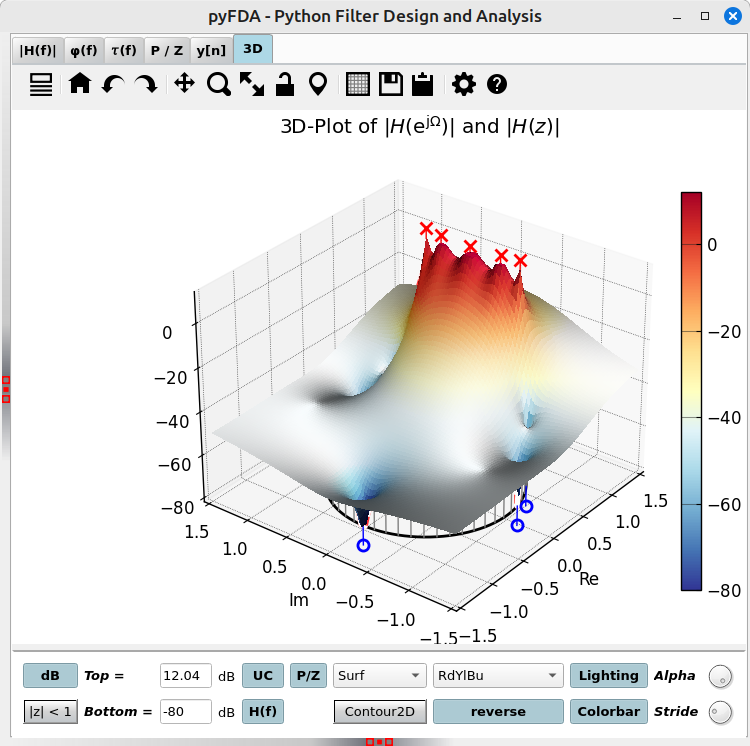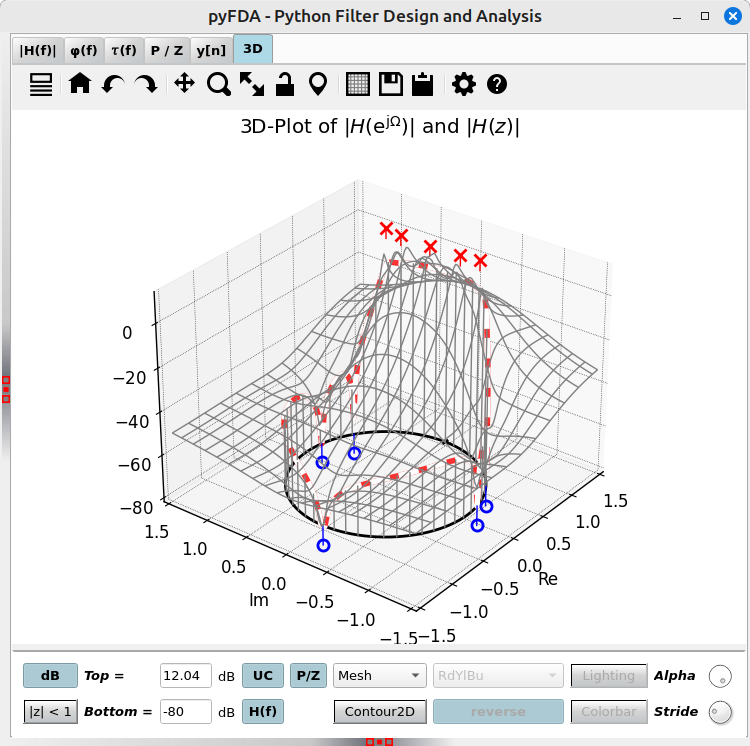# Plot 3D¶

Fig. 24 shows a typical view of the 3D tab for 3D visualizations of the magnitude frequency response and poles / zeros. Fig. 24 is a surface plot which looks nice but takes the longest time to compute.Fig. 24 Screenshot of the 3D tab (surface plot)

You can plot 3D visualizations of $$|H(z)|$$ as well as $$|H(e^{j\omega})|$$ along the unit circle (UC).

For faster visualizations, start with a mesh plot (Fig. 25) or a contour plot and switch to a surface plot when you are pleased with scale and view.Fig. 25 Screenshot of the 3D tab (mesh plot)